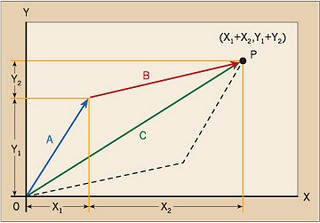# Notes of Vector AnalysisNotes of the vector analysis are given on this page. These notes are helpful for BSc or equivalent classes. These notes are written by Amir Taimur Mohmand of University of Peshawar. The books of these notes is not known. If you know about the book, please inform us.

Partial contents of these notes are given below. Notes covers the notions given below and related problems.

• Definition of vector
• Null or zero vector
• Magnitude of vectors
• Unit Vector
• Direction cosines
• Scalar product
• Law of cosine
• Vector (cross) product
• Scalar triple product (box product)
• Vector triple product
• Volume of parallelopiped
• Lagrange's identity
• Application of Vector Algebra
• Formula for Unit Vector
• Law of Cosine
• Altitudes of a triangle
• Work-done
• Vector moment
• Derivative of a vector function
• Differential equation
• Initial value problems
• Solution of differential problems
• List of formulas of differential operators
• Harmonic function
• Directional derivative
• Level surface
• Unit normal vectors
• The vectors differential operator
• Divergence of a vector
• Laplace operator
• Laplacian of a scalar function $f$
• Laplace's equation
• Harmonic function
• Gradient of a scalar function
• Directional derivative
• Level surfaces of a scalar function
• Unit normal vector to a level surface at point $P$
• Curl of a vector
• bsc/notes_of_vector_analysis